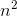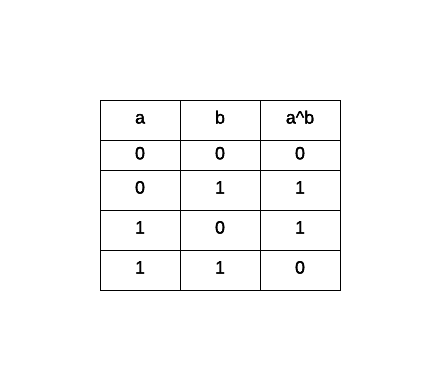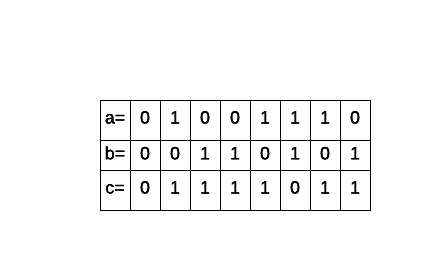Computer Science : Algorithm Analysis

Example Questions

2 Next →

Example Question #1 : Comparing Run Times

Which has faster compile time, O(N), O(N2), O(N3), or O(NlogN)?

O(N)

O(N2)

O(NlogN)

O(N3)

O(N)

Explanation:

O(NlogN) is O(N) * O(logN) which is greater than O(N) alone.

O(N2) is O(N*N) which is greater than O(N).

O(N3) is O(N*N*N) which is greater than O(N).

O(N) is the smallest and therefore is the quickest to compile. Therefore, O(N) is the correct answer.

Example Question #2 : Comparing Run Times

Which is more efficient?

a)

arr = [0, 1, 2, 3]

for (int i = 0; i < arr.length; i++) {

int j = 0;

if (j == arr[i]) {

j++;

}

}

b)

ArrayList<Integer> arL = new ArrayList<Integer>();

for (int i = 0; i < arL.size(); i++) {

int k = 0;

if (k == arL.get(i)) {

k++;

}

}

b)

Arrays are more efficient than ArrayLists

a)

a) and b) have the same efficiency

a) and b) have the same efficiency

Explanation:

The two code snippets have the same efficiency. Both operate in O(N) time. ArrayLists use arrays as their underlying data structure so access to the data is also the same.

Example Question #3 : Comparing Run Times

True or False.

The code snippet A has a more efficient running time than code snippet B.

(A)

for (int i = 0; i < 25; i++) {

for (int j = i; j < 25; j++) {

}

}

(B)

for (int i = 0; i < 25; i++ {

for (int j = 0; j < 25; j++) {

}

}

True

False

False

Explanation:

Code snippet A has a running time of O(N2). Code snippet B has a running time of O(N). While the two code snippets may look the same, the second for loop in code snippet A sets j=i. Since j is relying on i, it's multiplying the first for loop's running time by the second for loop's running time. This gives us O(N*N) or just O(N2).

Example Question #1 : Comparing Run Times

for( int i = 0; i < n; ++i){

for( int j = 1; j < n; j *= 2){

someFunction();

}

}

For the code above, what is the run time in Big O notation?

None of the above

O()

O( log(n) )

O( n )

O(n log(n) )

O(n log(n) )

Explanation:

At first glance we might be tempted to pick O() because there are 2 for loops. But, upon closer inspection we can see that the first loop will yield a O( n ) running time but the second loop does not. The second loop has only an O( log(n) ) running time because "j" doubles each iteration and does not increase linearly. That will yield O( log(n) ) since O( log(n) ) is a much faster running time. So the final result is O( n log(n) ).

BITWISE XOR OPERATION

Given the following binary values

a = 0100 1110

b = 0011 0101

perform an XOR operation (c = a^b). What is the result?

c = 0111 1011

c = 0000 0100

c = 0111 0011

c = 0111 1111

c = 0111 1011

Explanation:

Performing a bitwise excludive OR constitutes in taking both binary values and evaluating as follows: either one or the other (1) never both (0). This is the truth table for a bitwise XOR operation:Taking both a and b and performing the operation bit by bit we get the following result:Example Question #12 : Algorithm Analysis

True or False.

There is a runtime exception in this code snippet.

int wait_time = 0;

int wait_time = 5;

for (int i = 0; i < wait_time; i++) {

System.out.println(wait_time);

}

True

False